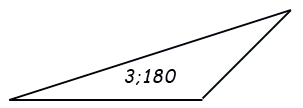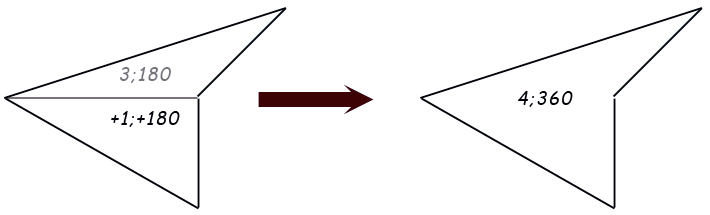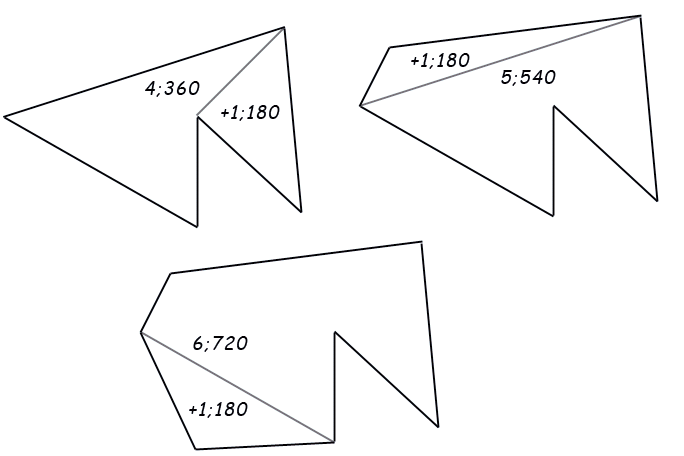SEARCH HOMEMath Central Quandaries & QueriesQuestion from Farzan, a student: Hello, Thanks a lot for all your last helps. This is my question : Prove with induction that in a polygon( that may not be convex ) with n sides, the sum of the amounts of the angles become 180(n-2). If there is any easier methods to prove the problem, please write as well.Farzan,

Here's the model of a proof.

Begin with a triangle.The sum of the interior angles makes 180 degrees.

Add another triangle externally to any one side.The sum of the new triangles interior angles is also 180. The sum of the interior angles of the polygon (ignoring internal lines) is 180 + the previous total. The number of external sides is 1 + the previous total.